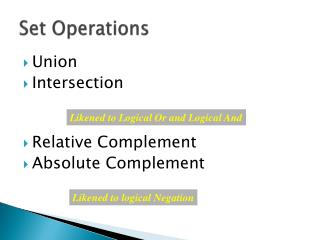DownloadDownload PresentationSet Operations

# Set Operations

Download Presentation## Set Operations

- - - - - - - - - - - - - - - - - - - - - - - - - - - E N D - - - - - - - - - - - - - - - - - - - - - - - - - - -
##### Presentation Transcript

1. Set Operations • Union • Intersection • Relative Complement • Absolute Complement Likened to Logical Or and Logical And Likened to logical Negation

2. Set Operations: Union of Sets • The union of two sets is the set that contains elements belonging to either of the two sets • Equivalent to the Boolean operation “or” • Written as: • Examples: A = {a, b, c, d} B = {c, d, e, f} A  B = {a, b, c, d, e, f} Note that the set could have been described as {a, b, c, d, c, d, e, f}

3. Union of Sets: Venn Diagrams A = {a, b, c, d} B = {c, d, e, f} A  B = {a, b, c, d, e, f} A = {a, b, c, d} B = {x, y, z} A  B = {a, b, c, d, x, y, z} Sets overlap Sets are disjoint

4. Set Operations: Set Intersection • The intersection of two sets is the set of all elements common to both sets • The intersection of disjoint sets is the empty set • Equivalent to the Boolean operation “and” • written as: • Examples: A = {a, b, c, d} A = {a, b, c, d} B = {a, b} B = {x, y, z} A  B = {a, b}A  B = 

5. Set Intersection : Venn Diagrams A = {a, b, c, d} B = {a, b} A  B = {a, b} A = {a, b, c, d} B = {x, y, z} A  B = 

6. Set Operations: Relative Complement • The relative complement (difference) of two sets is the set of elements contained in one, but not both, of the sets • Related to the Boolean “Exclusive Or” • Written as: — • Examples:Given:A = {a, b, c, d} and B = {a, c, f, g} A — B = {b, d} B — A = {f, g}

7. Relative Complement of Sets:Venn Diagrams A = {a, b, c, d} B = {a, c, f, g} A — B = {b, d} B — A = {f, g}

8. Absolute Set Complement • The absolute complement of a set is the set of elements which do not belong to the set being complemented’ • Equivalent to the Boolean operation “not” • Written as a superscripted ‘c’ • Example: U = {a, b, c, d, e, u, v, w, x, y, z} A = {a, b, c, x, y, z} and B = {a, b, c, d, e} Ac= {d, e, u, v, w} Bc = {u, v, w, x, y, z}

9. Absolute Complement of Sets:Venn Diagrams U = {a, b, c, d, e, u, v, w, x, y, z} A = {a, b, c, x, y, z} B = {d, e, y, z} Ac = {d, e, u, v, w}

10. Classic Boolean Model • Illustrates the 8 possible relations be-tween Sets, A, B and C

11. Membership Tables • Shows whether an arbitrary element x be- longs in any of the indicated sets.

12. Laws of the Algebra of Sets • Idempotent Laws A  A = A A  A = A • Associative Laws (A  B)  C = A  (B  C) (A  B)  C = A  (B  C) • Commutative Laws A  B = B  A A  B = B  A

13. … Laws of the Algebra of Sets • Distributive Laws A  (B  C) = (A  B)  (A  C) A  (B C) = (A  B)  (A  C) • Identity Laws A  = A A U = U A  U = A A   = 

14. …Laws of the Algebra of Sets • Complement LawsA  Ac = U Uc = A  Ac =   c = U • De Morgan’s Laws (A  B)c = Ac  Bc(A  B)c = Ac Bc